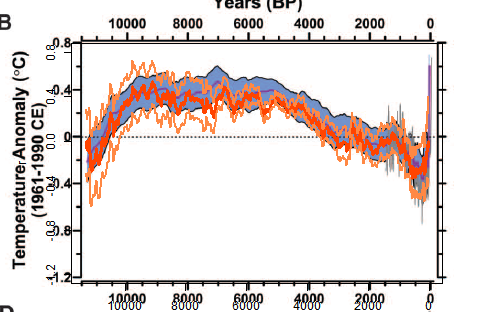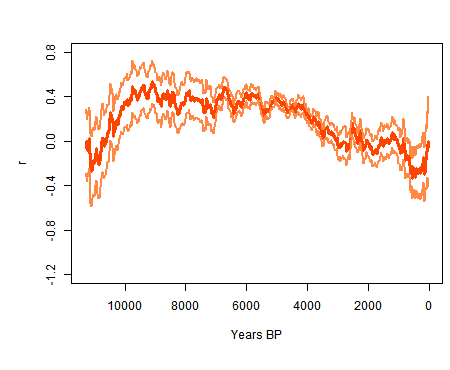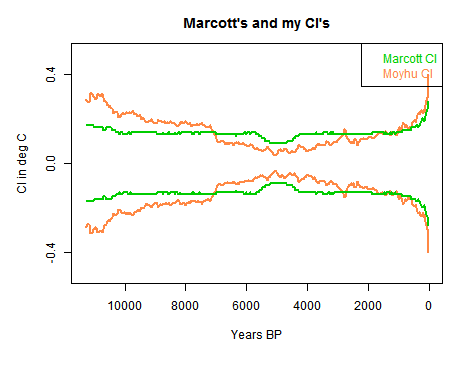## Saturday, April 13, 2013

### ## Confidence intervals match Marcott et al

In recent days there have been two issues raised at Climate Audit regarding the confidence intervals shown in the proxy reconstruction of Marcott et al. In the first, Romanm claimed that there was a major term missing from the confidence intervals, which could only be remedied by going back to the original calibration experiments and importing the variation of residuals as uncertainties in Marcott's analysis.

And in the second, an issue was made about a "dimple" in the confidence intervals, which in the two previous posts I have shown to be a perfectly natural consequence of the anomaly calculation.

Some weeks ago, I posted an early emulation, without the Monte Carlo variation of dating and calibration, of Marcott's analysis. In this post I will revisit that to show that the confidence intervals calculated purely on between proxy variation match those of Marcott, including the dip.

In Roman's thread, I argued strongly that the variation he said had been excluded was well covered by between proxy variation in the reduction of the 5x5 stack, and that this was the normal way of handling it. I cited Loehle and McCulloch, 2008 which had done this directly. This was apropos, because that paper had been discussed at Climate Audit prior to submission, and met with approval.

The counter was that the description of the stack reduction could be interpreted to say that the between-proxy variation had not been taken into account. I believed though that it must have been; the requirement to do so would have been obvious in the method.

So here I repeat the simple emulation, which is essentially the method of Loehle and Mcculloch. The code is at the end.

#### Emulation superimposed

In this plot, as in the earlier post, I am using grid weighting as im M13, and the "published dates", so the controversial spike is absent. The standard error of the mean is just the appropriate weighted variance, and this is used in the 1Ïƒ CI. The plot is as in my earlier post, except that I am now using the correct 4500:5500BP anomaly base. The CI's of my curve are shown in orange.Here is the curve and CI's in isolation.And here are the CI's shown without the curve, mine and Marcott's. My curve lacks the smoothing effect of Marcott's, but you can still see the dimple. But the main thing is that it matches well, and over the central range mine are narrower, despite the lack of smoothing. Marcott et al includes dating and calibration uncertainty.In the light of this, I am now confident that Marcott included between proxy variation in his CI calc.

#### Code

There is a zipfile ,a href="https://s3-us-west-1.amazonaws.com/www.moyhu.org/misc/peru/dimple.zip">here
with code and data.

# A program written by Nick Stokes www.moyhu.blogspot.com, to emulate a 5x5 recon from
#A Reconstruction of Regional and Global Temperature for the Past 11,300 Years
# Shaun A. Marcott, Jeremy D. Shakun, Peter U. Clark, and Alan C. Mix
#Science 8 March 2013: 339 (6124), 1198-1201. [DOI:10.1126/science.1228026]
#https://www.sciencemag.org/content/339/6124/1198.abstract

# Need this package:
getxls=T # set to T if you need to read the XL file directly
# The spreadsheet is at this URL "https://www.sciencemag.org/content/suppl/2013/03/07/339.6124.1198.DC1/Marcott.SM.database.S1.xlsx"
# Unfortunately it can't be downloaded and used directly because the R package can't read the format. You need to open it in Excel or OpenOffice and save in an older format.

loc="Marcott.SM.database.S1.xls"
prox=list()
if(getxls){
o=is.na(lat[,1])
lat=as.matrix(lat[!o,])
w=rep(0,36*72)
wu=1:73; k=1;
for(i in 1:73){
j=lat[i,]%/%5;
j=j+j*36+1297;
if(w[j]==0){w[j]=k;k=k+1;}
wu[i]=w[j];
}
# Read and linearly interpolate data in 20 yr intervals (from 1940)
m=11300/20
tm=1:m*20-10
g=list()
h=NULL
source("CI.txt")
if(getxls){
for(i in 1:73){
}
save(prox,lat,file="prox.sav")
}

for(i in 1:73){ # Anomalize
u=prox[[i]]
g[[i]]=approx(u[],u[],xout=tm) # R's linear int
y=g[[i]]\$y
y=y-mean(y[-25:25+250]) # anomaly to 5800-6200 BP
h=cbind(h,y)
}
r=rs=NULL # Will be the recon
# now the tricky 5x5 weighting - different for each time step
for(i in 1:nrow(h)){ # loop over time
o=!is.na(h[i,]) # marks the data this year
g=h[i,o];
u=wu[o]; n=match(u,u); a=table(n); d=as.numeric(names(a));
e=u;e[d]=a;wt=1/e[n]; #wt=weights
wt=wt/sum(wt)
# calc mean and variance
mn=sum(g*wt)
va=sum((wt*(g-mn))^2)
# Accumulate mean and CI
r=c(r,mn)
rs=c(rs,sqrt(va))
}
# Now standardize to 1961-90, but via using M et al value = -0.04 at 1950 BP
r=r-r-0.04
# now tricky graphics. my.png is the recon. ma.png is cut from fig 1b of the paper. You have to cut it exactly as shown to get the alignment right
ch="#ff8844"
png("myrec0.png",width=450,height=370)
par(bg="#ffffff00") # The transparent background
par(mar=c(6,5,3,0.5))
plot(tm,r,type="l",xlim=c(11300,0),ylim=c(-1.2,0.8),col="#ff4400",lwd=3,yaxp=c(-1.2,0.8,5))
lines(tm,r+rs,lwd=2,col=ch)
lines(tm,r-rs,lwd=2,col=ch)
dev.off()
png("myrec_0.png",width=450,height=370)
par(bg="#ffffff00") # The transparent background
par(mar=c(6,5,3,0.5))
plot(tm,r,type="n",xlim=c(11300,0),ylim=c(-0.5,0.5),col="#ff4400",lwd=3,yaxp=c(-1.2,0.8,5))
lines(tm,rs,lwd=2,col=ch)
lines(tm,-rs,lwd=2,col=ch)
lines(tm,un,lwd=2,col=3)
lines(tm,-un,lwd=2,col=3)
dev.off()
# Now an ImageMagick command to superpose the pngs
# You can skip this if you don't have IM (but you should)
system("composite myrec0.png ma.png reco0.png")